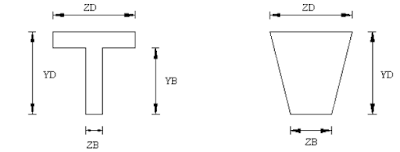## Wednesday, April 26, 2017

### How do i define Prismatic Properties in Staad?

The following prismatic properties are required for analysis:
• AX = Cross sectional area
• IX = Torsional constant
• IY = Moment of inertia about y-axis.
• IZ = Moment of inertia about z-axis.
In addition, the user may choose to specify the following properties:
• AY = Effective shear area for shear force parallel to local y-axis.
• AZ = Effective shear area for shear force parallel to local z-axis.
• YD = Depth of section parallel to local y-axis.
• ZD = Depth of section parallel to local z-axis.
For T-beams, YD, ZD, YB & ZB must be specified. These terms, which are shown
in the next figure are:
• YD = Total depth of section (top fiber of flange to bottom fiber of web)
• ZD = Width of flange
• YB = Depth of stem
• ZB = Width of stemPrismatic property nomenclature for a T and Trapezoidal section

For Trapezoidal beams, YD, ZD & ZB must be specified. These terms, which too
are shown in the next figure are:
• YD = Total depth of section
• ZD = Width of section at top fiber
• ZB = Width of section at bottom fiber
Top & bottom are defined as positive side of the local Z axis, and negative side of
the local Z axis respectively.
shear (in addition to deflection due to ordinary bending theory). To ignore the
shear deflection, enter a SET SHEAR command before the joint coordinates. This
will bring results close to textbook results.
The depths in the two major directions (YD and ZD) are used in the program to
calculate the section moduli. These are needed only to calculate member stresses
or to perform concrete design. You can omit the YD & ZD values if stresses or
design of these members are of no interest.
To define a concrete member,you must not provide AX, but instead, provide YD
and ZD for a rectangular section and just YD for a circular section. If no moment
of inertia or shear areas are provided, the program will automatically calculate
these from YD and ZD.Required Section Properties

Table is offered to assist the user in specifying the necessary section values. It
lists, by structural type, the required section properties for any analysis. For the
PLANE or FLOOR type analyses, the choice of the required moment of inertia
depends upon the beta angle. If BETA equals zero, the required property is IZ.